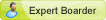## Plottable cloud base heights calculated by a virtual sensor

Moderator: Mattk

d_l
Expert BoarderPosts: 138
Joined: Fri Mar 13, 2009 12:58 am
Contact:

### Plottable cloud base heights calculated by a virtual sensor

While the minimum cloud base is calculated by the Meteohub and can be displayed as text by the [actual_th0_cloudheight_f] name-pair, this ouput is not readily plottable (AFAIK). If you wish to plot cloud base heights over a period of time, you will need to make a virtual sensor to produce that data and then plot those results.

This cloud base AWK script is fairly simple. However, the AWK/GAWK manuals do not address the immensely significant fact that the Meteohub's internal calculations are computed at full values and then divided by 100 before being displayed as a Meteohub sensor value. This virtual sensor is also programmed to round up values greater or equal than x.5 and round down those below to yield a "decimal integer".

First of all, this computation is still using Meteohub version 4.6t (I don't update often), but I think it should run correctly on later versions.

These are the "how-to" settings:

For your virtual sensor, define the following:
New ID - data#
Name - CB or what ever you wish
Trigger - th#
Conversion - awk ' { cb = ((\$3/10 - \$5/10)/2.5)* 1000; cb = int (cb -- 0.5) * 100; printf ''%i", cb } '

Simply copy and paste the AWK script into the conversion box in order to avoid entry mistakes.

The cloud base formula for height in feet is CB = (T – Tdew) / 2.5 * 1000. Note that the temperature value as output by the Meteohub must be divided by 10 and the overall height multiplied by 100 to produce the correct cloud base height. If you wish the height to be in meters, substitute 4.4 for 2.5 in the script. The AWK script actually calculates the height above the station's temp/hum sensor which is often stated as above ground level (AGL). For the actual elevation of cloud bases, the temp/hum sensor's elevation has to be added to the AWK script. If you wish the script output to be the actual elevation of a cloud base, I suggest adding that elevation right after the 100 in the script. Remember that to add in the Meteohub AWK scripts, you need to use a double negative such as "-- elevation value".

For now, I've been plotting the cloud base height on a simple 24-hr graph - https://www.tceweather.com/cloudbase_graph.html. This page is still a work-in-progress as I'm unsure of the maximum height that the graph's Y-axis will need. The graph's Format Cmd string is "set term png transparent 9|set lmargin 10|set xzeroaxis lt 2 lw 2|set format y "%.0f"|set ylabel "Cloud Base (ft)"|set format y2 "%.0f"|set y2label "Cloud Base (ft)"|set timestamp top "%m/%d/%y %H:%M" offset 5, -2|set key box at graph 1.05,1.06 horizontal". I generally like to have transparent plots.

Edit: The changes in the cloud base height over time look almost identical to the Revised Chandler Burning Index when graphed (except when the cloud heights exceed about 10k ft, the CBI flatlines at the 100 cap). Both use T and RH (the RH for the cloud base is indirect through the dew point), so similar curves would be expected.